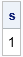A SAS/IML programmer asked a question on a discussion forum, which I paraphrase below:

I've written a SAS/IML function that takes several arguments. Some of the arguments have default values. When the module is called, I want to compute some quantity, but I only want to compute it for the parameters that are supplied and are nonempty.

To illustrate the programmer's dilemma, consider the following SAS/IML function:

```proc iml; start Func1(a, b=2, c=); sum = a + b + c; /* WRONG */ return( sum ); finish;```

This function uses optional parameters. The function is supposed to return the sum of three scalar arguments, but there is a problem: the addition operator will not add an empty matrix. (Recall that an empty matrix has no rows or columns.) If the second parameter is supplied but is empty, then the addition operation will fail. Similarly, if the third parameter is skipped, then the default value of c is an empty matrix, and the addition operation also fails. The programmer was asking how to compute the sum over only those parameters that are supplied and are nonempty.

You can detect which parameters are supplied by calling the ISSKIPPED function from within the module. The ISSKIPPED function indicates whether an argument was provided to the module or whether it was not provided (was skipped). The argument to the ISSKIPPED function must be one of the argument names.

The ISSKIPPED function will return 0 (FALSE) if the parameter was passed in, even if the value of the parameter is an empty matrix. However, you can use the ISEMPTY function to detect an empty matrix.

Consequently, the following function computes the sum of the parameters that are supplied and are nonempty:

```start Func(a, b=2, c=); /* compute sum of parameters that are supplied */ sum = 0; if ^isskipped(a) & ^isempty(a) then sum = sum + a; if ^isskipped(b) & ^isempty(b) then sum = sum + b; if ^isskipped(c) & ^isempty(c) then sum = sum + c; return( sum ); finish;   s = Func(1, NULL); /* NULL is an undefined (empty) matrix */ print s;```The second parameter is not included in the sum because it is an empty matrix. The third parameter is not included in the sum because it was not supplied.

This example is somewhat contrived, but it does show how to use the ISSKIPPED and ISEMPTY functions to detect two properties of arguments to a module.

Share